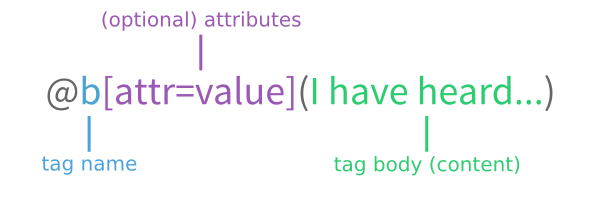# Scriba » Reference

Below is the complete reference to the Scriba format.

# BasicsThis is a begin/end block:

``````@begin(tag)
content
@end(tag)
``````

Paragraphs don't have to be explicitly marked up, they are automatically determined by two newlines. That is, the following:

``````This is a paragraph

This is another paragraph.
``````

Will produce:

This is a paragraph

This is another paragraph.

# Formatting

The following tags provide basic formatting:

`@b`
Bold text.
`@i`
Italicized text.
`@u`
Underlined text.
`@strike`
Striked-out text.
`@c`
Inline code.
`@sup`
Superscripted text.
`@sub`
Subscripted text.

For instance, the following:

``````This text is @b(bold), this is @i(italics), and this is @c(code). You might also
want @u(underlined) text.

The speed of light is 3x10@sup(8) meters per second. The symbol for the
permittivity of vacuum is ε@sub(0).
``````

Will produce the following:

This text is bold, this is italics, and this is `code`. You might also want underlined text.

The speed of light is 3x108 meters per second. The symbol for the permittivity of vacuum is ε0.

# Sections

Unlike HTML and Markdown, where sections are implicit in the positions of headings, CommonDoc (and, thus, Scriba) makes sections explicit blocks.

``````@begin(section)
@title(Section)

@begin(section)
@title(Subsection)

@begin(section)
@title(Sub-subsection)

@end(section)

@end(section)

@begin[ref=section-id](section)
@title(Another subsection)

@end(section)

@end(section)
``````

The indentation above is optional, normally, section tags are flat.

# Lists

Scriba provides three types of lists: unordered, enumerated, and definition lists.

## Unordered

Unordered lists can be created with the `@list` tag. Individual list items go in `@item` tags.

``````@begin(list)
@item(An item.)
@item(In @i(a) list.)
@end(list)
``````

This will produce the following:

• An item.
• In a list.

## Enumerated

Enumerated lists can be created with the `@enum` tag. As in unordered lists, list items use `@item` tags.

``````@begin(enum)
@item(The first item.)
@item(The second item.)
@item(Third.)
@end(enum)
``````

Will produce this:

1. The first item.
2. The second item.
3. Third.

## Definition

Definition lists can be created with the `@deflist` tag. Inside the tag there are alternating `@term` and `@def` tags. For instance, the markup below:

``````@begin(deflist)

@term(VerTeX)
@def(A markup language with text syntax.)

@term(Scriba)
@begin(def)
A markup language with Scribe syntax.

As you can see, you can use regular tags and begin/end ones interchangeably.
@end(def)

@end(deflist)
``````

Will produce this:

VerTeX
A markup language with text syntax.
Scriba

A markup language with Scribe syntax.

As you can see, you can use regular tags and begin/end ones interchangeably.

# Code

The `lang` attribute is a string identifying the language of the code. No restrictions are placed on this value.

The following markup:

``````@begin[lang=lisp](code)
(defun fact (n)
(if (= n 1)
1
(* n (fact (1- n)))))
@end(code)
``````

Will produce:

``````(defun fact (n)
(if (= n 1)
1
(* n (fact (1- n)))))
``````

You can also use the include contrib to include code from a file, or a subrange within a file.

## Internal

The following markup:

``````@begin[ref=data-table-3](table)
@row(@cell(@b(A)) @cell(@b(B)) @cell(@b(C)))
@row(@cell(1.23) @cell(3.14) @cell(4.5))
@end(table)

...

See the third @ref[id=data-table-3](table) for the data.
``````

Will produce the following table and link:

 A B C 1.23 3.14 4.5

...

See the third table for the data.

You can also link to nodes inside other documents, where a document is identified by a string:

``````For a more thorough discussion, see @ref[doc=aima id=search](the AIMA chapter)
on search algorithms.
``````

## External

You can link to external URLs using the `@link` tag. This:

``````Visit @link[uri="https://www.google.com/"](Google).
``````

Will produce:

# Images & Figures

You can link to images in the filesystem relative to the document:

``````@image[src=path/to/image.png]()
``````

Or to images on the web. For instance, a Travis build status badge:

``````@image[src="https://travis-ci.org/eudoxia0/crane.svg?branch=master"]()
``````

will produce:# Tables

``````@begin(table)
@begin(row)
@cell()
@cell(@b(Peach))
@cell(@b(Egg))
@end(row)
@begin(row)
@cell(@i(Fat))
@cell(0.25g)
@cell(10.6g)
@end(row)
@begin(row)
@cell(@i(Protein))
@cell(0.91g)
@cell(12.6g)
@end(row)
@end(table)
``````

Produces the following:

 Peach Egg Fat 0.25g 10.6g Protein 0.91g 12.6g

Note that the indentation in this example is completely optional.